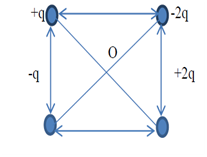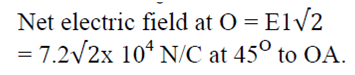## Sunday, 20 March 2016

### WORKSHET#3 ELECTRIC FIELD CBSE CLASS 12 PHYSICS QUESTIONS WITH KEY

1.Two point charges, q =8×10-8 C and Q= -2×10-8 C are separated by a distance of 10 cm in air. What is the net electric field at the mid- point between the charges.

Using the formulae=F/Q =3.6x 105

2. Two point charges 4 Q, Q are separated by 1 m in air. At what point on the line joining the charges is the electric field intensity zero?  Q9
x=2/3 m
3 .Two point charges of 16μC and -9μC are placed 8cm apart in air. Determine the position of the point at which resultant electric field is zero.
4 .Eight identical point charges of q Coulomb each are placed at corners of a cube of each side 0.1m .Calculate electric field at the center of the cube. Calculate the field at the center when one of the corner charges is removed.
i) zero ii) E = (1.2 x 1012)q N/C

5. What are the magnitude and direction of electric field at the center of the square in fig if q=1.0 x 10 -8C and a = 5cm?6. A charge of 4 x 10 -9 C is distributed uniformly over the circumference of a conducting ring of radius 0.3m. Calculate the field intensity at a point on the axis of the ring at 0.4m from its centre, also at the center.

At the center of ring, r = 0,
So E = 0
7. Charge q1=+6.0nC is on y axis at y=+3 cm and charge q2 = -6nCis on y axis at y=-3cm .Calculate force on test charge q0= 2 nC placed on x axis at x=4cm.

-51.8 j μN

8.ABC is an equilateral triangle of each side 5 cm. two charges of ±50/3 x 10-3 μC are placed at A and B respectively. Calculate magnitude and direction of resultant intensity at C.

6 x 104 N/C parallel to AB from + charge to – charge.